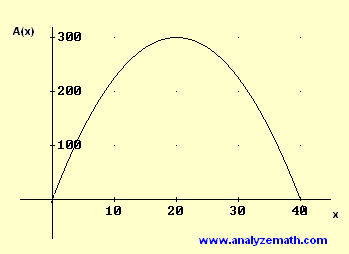# Maximum Area of Rectangle in a Right Triangle - Problem with Solution

Maximize the area of a rectangle inscribed in right triangle using the first derivative. The problem and its solution are presented

## Problem with Solution

BDEF is a rectangle inscribed in the right triangle ABC whose side lengths are 40 and 30. Find the dimemsions of the rectangle BDEF so that its area is maximum.Solution to Problem:

• let the length BF of the rectangle be y and the width BD be x. The area of the right triangle is given by (1/2)*40*30 = 600. But the area of the right triangle may also be calculated as the sum of the areas of triangle BEC and BEA. Hence
600 = (1/2)*40*y + (1/2)*30*x
• Let A be the area of the rectangle. Hence
A = y*x
• We now use the first equation to express y in terms of x as follows
y = (600 - 15x) / 20
• Substitute in A to obtain
A(x) = x(600 - 15x) / 20
• The graph of A(x) as a function of x is shown below. A(x) has a maximum value for x = 20. This will be shown analytically as well• An expansion of A(x) shows that A(x) is a quadratic function with negative leading coefficient and therefore has a maximum value.
A(x) = -(3/4)x2 + 30x
• We now calculate the first derivative of A.
A'(x) = -(3/2)x + 30
• Set A'(x) = 0 and solve for x.
x = 20
• It is easy to check that A'(x) is positive for x < 20 and negative for x > 20 and therefore A(x) has a maximum at x = 20. The maximum area is given by A(20)
A(20) = -(3/4)202 + 30*20 = 300
• We now find y as follows
A = 300 = x * y , y = 300/20 = 15
• The dimensions of the rectangle that make its area maximum are x= 20 and y = 15.

More references on calculus problems